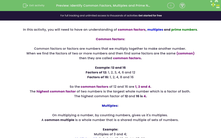# Identify Common Factors, Multiples and Prime Numbers

In this worksheet, students will use their knowledge of common factors, multiples and prime numbers to answer a variety of questions.Key stage:  KS 2

Curriculum topic:   Number: Addition, Subtraction, Multiplication and Division

Curriculum subtopic:   Identify Common Factors, Multiples and Prime Numbers

Popular topics:   Prime Numbers worksheets, Factors worksheets

Difficulty level:#### Worksheet Overview

In this activity, we will be finding common factors, multiples and prime numbers.

Common Factors

Factors are numbers that we multiply together to make another number.

When we find the factors of two or more numbers that are the same, then they are called common factors.

Example: 12 and 16

Factors of 12: 1, 2, 3, 4, 6 and 12

Factors of 16: 1, 2, 4, 8 and 16

The common factors of 12 and 16 are 1, 2 and 4.

The highest common factor of two numbers is the largest whole number which is a factor of both.

The highest common factor of 12 and 16 is 4.Multiples

The multiples of a number are the numbers that form its times table.

A common multiple is a whole number that is a shared multiple of two or more sets of numbers.

Example

Multiples of 3 and 4:

Multiples of 3: 3, 6, 9, 12, 15, 18, 21, 24, 27, 30

Multiples of 4: 4, 8, 12, 16, 20, 24, 28, 32, 36, 40

The common multiples of 3 and 4 are: 12 and 24.Prime Numbers

A prime number is a number only divisible by itself and 1.

So, prime numbers have only two factors.

Example

What are the prime numbers between 10 and 30?

Answer: 11, 13, 17, 19, 23, 29

These numbers have no factors except for the number 1 and the number itself.

That's a lot to remember, but it will become easier the more we practise.

Let's get started!

### What is EdPlace?

We're your National Curriculum aligned online education content provider helping each child succeed in English, maths and science from year 1 to GCSE. With an EdPlace account you’ll be able to track and measure progress, helping each child achieve their best. We build confidence and attainment by personalising each child’s learning at a level that suits them.

Get started